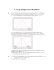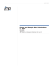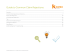# Hypothesis Testing Series (One Sample) STA2023-4a

## Transcription

Hypothesis Testing Series (One Sample) STA2023-4a
```STA2023-4a
Hypothesis Testing Series (One Sample)
Case 1: Testing a Claim About a Mean 𝛍: Large Sample (N>30)
A.
REJECTION REGION METHOD
1.
State the Null Hypothesis H0
This is a hypothesis that must contain a statement of equality, such as ≤, =, or ≥
2.
State the Alternative Hypothesis Ha (some texts use H1)
This is the complement of the null hypothesis; i.e., it must be true if H0 is false.
This hypothesis must contain a statement of inequality, such as <, ≠, or >.
3.
Specify the Level of Significance Alpha (𝛂)
The probability of rejecting the null hypothesis when it is true.
4.
Decide whether the test is left-tailed, right-tailed, or two-tailed.
If the alternative hypothesis contains:
<
it is a left-tailed test
≠
it is a two-tailed test
>
it is a right-tailed test
HINT: The inequality symbols ‘<’ and ‘>’ point to the rejection region.
5.
Find the Critical Value(s)
This is the value that separates the rejection region from the non-rejection region.
It is a z score that for a



left-tailed test is the negative z score that corresponds to an area of ∝
right-tailed test is the positive z score that corresponds to an area of
1-∝
two-tailed test are the two z scores that correspond to the areas of ½ ∝
and 1 - ½ ∝
SOME COMMON CRITICAL Z SCORES
Alpha (∝)
Tails
Critical Z
.05
2
LEFT
RIGHT
+1.96, -1.96
-1.645
+1.645
.01
2
LEFT
RIGHT
+2.58, -2.58
-2.33
+2.33
Testing a Claim About a Mean 𝛍: (Large Sample)
1
Document created by South Campus Library Learning Commons 03/26/10. Permission to copy and use is
granted for educational use provided this copyright label is displayed.
STA2023-4a
6.
Sketch the Normal Distribution
Add the critical value(s), and shade in the corresponding rejection region. Below
is an example for a two-tailed test.
7.
Calculate the Test Statistic
̅
⁄
√
If σ is unknown, use s as an approximation
Determine where Z is located in relation to the rejection region identified in the
previous step.
8.
Make a Decision
If Z is in the rejection region, make a decision to „Reject H0‟. If Z is outside the
rejection region, make a decision to „Fail to Reject H0‟.
9.
Restate the Decision in Non- Technical Terms
If the original claim contained the condition of equality (
≤ ≥), and:
a. you failed to reject H0, it should be stated similar to the following: “There is
not sufficient evidence to warrant rejection of the claim that … (original
claim)”
b. you rejected H0, it should be stated similar to the following “There is sufficient
evidence to warrant rejection of the claim that … (original claim)”
If the original claim contained the condition of inequality ( ≠
), and:
a. you rejected H0, it should be stated similar to the following: “The sample data
support the claim that … ( original claim).”
b. you failed to reject H0, it should be stated similar to the following “There is not
sufficient sample evidence to support the claim that … (original claim)”
Testing a Claim About a Mean 𝛍: (Large Sample)
2
Document created by South Campus Library Learning Commons 03/26/10. Permission to copy and use is
granted for educational use provided this copyright label is displayed.
STA2023-4a
B.
P- VALUE METHOD
The steps to conduct the hypothesis test for the mean using P-values are similar
to the traditional method previously discussed, with the main difference that we
will be comparing our P-value to the level of significance rather that comparing a
test statistic to a rejection region. The test statistic is still required, however, since
it determines the size of the tail, which in turns determines the value of P.
1.
State the Null Hypothesis H0
2.
State the Alternative Hypothesis Ha (some texts use H1)
3.
Specify the level of significance Alpha (𝛂)
4.
Decide whether the test is left-tailed, right-tailed, or two-tailed.
5.
Calculate the Test Statistic (Use Z because n>30)
̅
if σ is unknown, then use s as an approximation of σ
√
6.
Find the P-value



7.
For a left-tailed test:
For a right-tailed test:
For a two-tailed test:
P = Area to the left of the test statistic
P = Area to the right of the test statistic
P = twice the area in the tail beyond the test
statistic.
Make a decision to Reject or Fail to Reject H0
Reject H0 if the P-value ≤ α. Otherwise, fail to reject H0
8.
Restate the Decision in Non- Technical Terms
Refer to Step 9 of the previous section (Rejection Region Method) for acceptable
verbiage.
Testing a Claim About a Mean 𝛍: (Large Sample)
3
Document created by South Campus Library Learning Commons 03/26/10. Permission to copy and use is
granted for educational use provided this copyright label is displayed.
STA2023-4a
C.
TI-83/84 METHOD
1.
Determine the Null Hypothesis H0, Alternative Hypothesis Ha, and
Significance Level α
2.
Press STATS
3.
Select TESTS, then choose first option of Z-Test
a)
b)
c)
Input:
Data (Use if your data resides in lists on the calculator) or
Stats (Use if you just have summary stats such as σ and µ)
Enter Null Hypothesis value µ0
Enter σ
Remember: If σ is unknown (as it usually is), and the sample size is
large, use the value of s as a reasonable approximation of σ
d)
e)
Enter sample mean ̅ and sample size n
Enter alternative hypothesis (µ≠µ0; µ< µ0; µ> µ0)
f)
Press Calculate
Interpretation from above TI-83/84 screen shots:
1. P test:
P=.5481
If α is .05, then P>α, which means do not reject the null.
2. Rejection Region Method: For two-tailed test, the critical values are ±1.96
significance level of .05. Since z = -0.6007 is between -1.96 and +1.96 (zero
is in the middle), it is not in the rejection region, so do not reject the null.
Testing a Claim About a Mean 𝛍: (Large Sample)
4
Document created by South Campus Library Learning Commons 03/26/10. Permission to copy and use is
granted for educational use provided this copyright label is displayed.
STA2023-4a
Sample Exercise
Test the claim that µ = 40 given a sample of n = 75 for which ̅ = 39.2 and s = 3.23. Use a
significance level of .05
Rejection Region Method
P- Value Method
TI-83/84 Method
Step 1: Null: µ = 40
Null: µ = 40
Null: µ = 40
Step 2: Alternative: µ ≠ 40
Alternative: µ ≠ 40
Alternative: µ ≠ 40
Step 3: α = .05
α = .05
α = .05
Step 4: Critical Values: Z = ±1.96
Test Statistic
Press STATS
Select TESTS, then choose first
option Z- Test
̅
√
Input:
√
-1.96
+1.96
Step 5: Test Statistic
̅
√
√
= - 2.145
Step 6: Since Z= -2.145 is in the
rejection region (< -1.96; see Step
4), the decision is to reject the
null.
= - 2.145
Stats
µ0 = 40
σ = 3.23
̅
n = 75
µ ≠ µ0
For a two-tailed test, P is
twice the area in the tail of
the test statistic.
Z = -2.145 corresponds to a
tail of 0.0158.
Therefore P= 2(.0158)
=0.0316
Since P < α (.0316 < .050),
the decision is to reject the
null.
Press ‘Calculate’:
Reject the null for one of two
reasons:
1. P<.05 (.032 < .05)
2. Z= -2.145, which is less
than -1.96 (therefore it is
in the rejection region)
Testing a Claim About a Mean 𝛍: (Large Sample)
5
Document created by South Campus Library Learning Commons 03/26/10. Permission to copy and use is
granted for educational use provided this copyright label is displayed.
```

### Document 6537334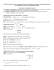### Inferential Statistics t and ScWk 242 – Session 9 Slides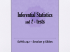### Stat 100 Sample Final Questions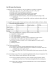### Sections 8-1 and 8-2 - Gordon State College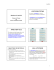### chapter 9 practice - faculty.piercecollege.edu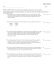### STAT 2000 Sample Midterm (actually given in October 2007)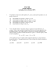### Chapter 7 Lecture Notes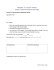### Dear Ms. Jensen and Mr. Maxwell Please accept this claim in the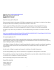### What is Hypothesis Testing? AP Statistics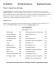### Sampling Distributions and One Sample Tests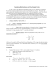### Math 119 Sample Final Exam Open book and note Calculator OK Multiple Choice  1 point each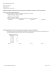### Using Your TI-NSpire Calculator for Hypothesis Testing: t Dr. Laura Schultz Statistics I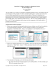### Chapter 8 practice - faculty.piercecollege.edu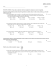### 7.6 Large-Sample Test of Hypothesis about a Population p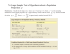### 1 Pop Quiz: This quiz is worth 85% of your final grade. You have 2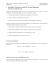### Problem Set 5, ECON 30331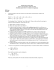### Chapter 11 Introduction to Hypothesis Testing 11.1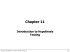### 9: Large-Sample Tests of Hypotheses ( )